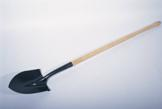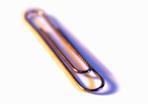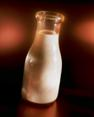## Metric System Basics

### Learning Outcomes

• Describe the general relationship between the U.S. customary units and metric units of length, weight/mass, and volume
• Define the metric prefixes and use them to perform basic conversions among metric units
• Solve application problems using metric units
• State the freezing and boiling points of water on the Celsius and Fahrenheit temperature scales.
• Convert from one temperature scale to the other, using conversion formulas

## What Is Metric?

The metric system uses units such as meter, liter, and gram to measure length, liquid volume, and mass, just as the U.S. customary system uses feet, quarts, and ounces to measure these.

In addition to the difference in the basic units, the metric system is based on 10s, and different measures for length include kilometer, meter, decimeter, centimeter, and millimeter. Notice that the word meter is part of all of these units.

The metric system also applies the idea that units within the system get larger or smaller by a power of 10. This means that a meter is 100 times larger than a centimeter, and a kilogram is 1,000 times heavier than a gram. You will explore this idea a bit later. For now, notice how this idea of getting bigger or smaller by 10 is very different than the relationship between units in the U.S. customary system, where 3 feet equals 1 yard, and 16 ounces equals 1 pound.

## Length, Mass, and Volume

The table below shows the basic units of the metric system. Note that the names of all metric units follow from these three basic units.

 Length Mass Volume basic units meter gram liter other units you may see kilometer kilogram dekaliter centimeter centigram centiliter millimeter milligram milliliter

In the metric system, the basic unit of length is the meter. A meter is slightly larger than a yardstick, or just over three feet.

The basic metric unit of mass is the gram. A regular-sized paperclip has a mass of about 1 gram.

Among scientists, one gram is defined as the mass of water that would fill a 1-centimeter cube. You may notice that the word mass is used here instead of weight. In the sciences and technical fields, a distinction is made between weight and mass. Weight is a measure of the pull of gravity on an object. For this reason, an objects weight would be different if it was weighed on Earth or on the moon because of the difference in the gravitational forces. However, the objects mass would remain the same in both places because mass measures the amount of substance in an object. As long as you are planning on only measuring objects on Earth, you can use mass/weight fairly interchangeablybut it is worth noting that there is a difference!

Finally, the basic metric unit of volume is the liter. A liter is slightly larger than a quart.The handle of a shovel is about 1 meter. A paperclip weighs about 1 gram. A medium-sized container of milk is about 1 liter.

Though it is rarely necessary to convert between the customary and metric systems, sometimes it helps to have a mental image of how large or small some units are. The table below shows the relationship between some common units in both systems.

 Common Measurements in Customary and Metric Systems Length 1 centimeter is a little less than half an inch. 1.6 kilometers is about 1 mile. 1 meter is about 3 inches longer than 1 yard. Mass 1 kilogram is a little more than 2 pounds. 28 grams is about the same as 1 ounce. Volume 1 liter is a little more than 1 quart. 4 liters is a little more than 1 gallon.

## Prefixes in the Metric System

The metric system is a base 10 system. This means that each successive unit is 10 times larger than the previous one.

The names of metric units are formed by adding a prefix to the basic unit of measurement. To tell how large or small a unit is, you look at the prefix. To tell whether the unit is measuring length, mass, or volume, you look at the base.

 Prefixes in the Metric System kilo- hecto- deka- meter gram liter deci- centi- milli- 1,000 times larger than base unit 100 times larger than base unit 10 times larger than base unit base units 10 times smaller than base unit 100 times smaller than base unit 1,000 times smaller than base unit

Using this table as a reference, you can see the following:

• A kilogram is 1,000 times larger than one gram (so 1 kilogram = 1,000 grams).
• A centimeter is 100 times smaller than one meter (so 1 meter = 100 centimeters).
• A dekaliter is 10 times larger than one liter (so 1 dekaliter = 10 liters).

Here is a similar table that just shows the metric units of measurement for mass, along with their size relative to 1 gram (the base unit). The common abbreviations for these metric units have been included as well.

 Measuring Mass in the Metric System kilogram (kg) hectogram (hg) dekagram (dag) gram (g) decigram (dg) centigram (cg) milligram (mg) 1,000 grams 100 grams 10 grams gram 0.1 gram 0.01 gram 0.001 gram

Since the prefixes remain constant through the metric system, you could create similar charts for length and volume. The prefixes have the same meanings whether they are attached to the units of length (meter), mass (gram), or volume (liter).

### Try It

Which of the following sets of three units are all metric measurements of length?

A) inch, foot, yard

B) kilometer, centimeter, millimeter

C) kilogram, gram, centigram

D) kilometer, foot, decimeter

## Converting Units Up and Down the Metric Scale

Converting between metric units of measure requires knowledge of the metric prefixes and an understanding of the decimal systemthats about it.

For instance, you can figure out how many centigrams are in one dekagram by using the table above. One dekagram is larger than one centigram, so you expect that one dekagram will equal many centigrams.

In the table, each unit is 10 times larger than the one to its immediate right. This means that 1 dekagram = 10 grams; 10 grams = 100 decigrams; and 100 decigrams = 1,000 centigrams. So, 1 dekagram = 1,000 centigrams.

### Example

How many milligrams are in one decigram?

### Try It

Convert 3,085 milligrams to grams.

### Example

Convert 1 centimeter to kilometers.

### Try It

Once you begin to understand the metric system, you can use a shortcut to convert among different metric units. The size of metric units increases tenfold as you go up the metric scale. The decimal system works the same way: a tenth is 10 times larger than a hundredth; a hundredth is 10 times larger than a thousandth, etc. By applying what you know about decimals to the metric system, converting among units is as simple as moving decimal points.

Here is the first problem from above: How many milligrams are in one decigram? You can recreate the order of the metric units as shown below:

$\displaystyle kg\quad hg\quad dag\quad g\quad d\underbrace{g\quad c}_{1}\underbrace{g\quad m}_{2}g$

This question asks you to start with 1 decigram and convert that to milligrams. As shown above, milligrams is two places to the right of decigrams. You can just move the decimal point two places to the right to convert decigrams to milligrams: $\displaystyle 1\ dg=1\underbrace{0}_{1}\underbrace{0}_{2}.\ mg$.

The same method works when you are converting from a smaller to a larger unit, as in the problem: Convert 1 centimeter to kilometers.

$\displaystyle k\underbrace{m\quad h}_{5}\underbrace{m\quad d}_{4}\underbrace{am\quad }_{3}\underbrace{m\quad d}_{2}\underbrace{m\quad c}_{1}m\quad mm$

Note that instead of moving to the right, you are now moving to the leftso the decimal point must do the same:

$\displaystyle 1\ cm=0.\underbrace{0}_{5}\underbrace{0}_{4}\underbrace{0}_{3}\underbrace{0}_{2}\underbrace{1}_{1}\ km$.

### Try It

How many milliliters are in 1 liter?

## Factor Label Method

There is yet another method that you can use to convert metric measurementsthe factor label method. You used this method when you were converting measurement units within the U.S. customary system.

The factor label method works the same in the metric system; it relies on the use of unit fractions and the cancelling of intermediate units. The table below shows some of the unit equivalents and unit fractions for length in the metric system. (You should notice that all of the unit fractions contain a factor of 10. Remember that the metric system is based on the notion that each unit is 10 times larger than the one that came before it.)

Also, notice that two new prefixes have been added here: mega- (which is very big) and micro- (which is very small).

 Unit Equivalents Conversion Factors 1 meter = 1,000,000 micrometers $\displaystyle \frac{1\ m}{1,000,000\ \mu m}$ $\displaystyle \frac{1,000,000\ \mu m}{1\ m}$ 1 meter = 1,000 millimeters $\displaystyle \frac{1\ m}{1,000\ mm}$ $\displaystyle \frac{1,000\ mm}{1\ m}$ 1 meter = 100 centimeters $\displaystyle \frac{1\ m}{100\ cm}$ $\displaystyle \frac{100\ cm}{1\ m}$ 1 meter = 10 decimeters $\displaystyle \frac{1\ m}{10\ dm}$ $\displaystyle \frac{10\ dm}{1\ m}$ 1 dekameter = 10 meters $\displaystyle \frac{1\ dam}{10\ m}$ $\displaystyle \frac{10\ m}{1\ dam}$ 1 hectometer = 100 meters $\displaystyle \frac{1\ hm}{100\ m}$ $\displaystyle \frac{100\ m}{1\ hm}$ 1 kilometer = 1,000 meters $\displaystyle \frac{1\ km}{1,000\ m}$ $\displaystyle \frac{1,000\ m}{1\ km}$ 1 megameter = 1,000,000 meters $\displaystyle \frac{1\ Mm}{1,000,000\ m}$ $\displaystyle \frac{1,000,000\ m}{1\ Mm}$

When applying the factor label method in the metric system, be sure to check that you are not skipping over any intermediate units of measurement!

### Example

Convert 7,225 centimeters to meters.

### Try It

Convert 32.5 kilometers to meters.

Now that you have seen how to convert among metric measurements in multiple ways, let’s revisit the problem posed earlier.

### Example

If you have a prescription for 5,000 mg of medicine, and upon getting it filled, the dosage reads 5g of medicine, did the pharmacist make a mistake?